Homework Help Question & Answers

Exercise 1. We are solving the ODE yycos)1) 1, with a time step h 0.1. Calculate y at t-1.1 using...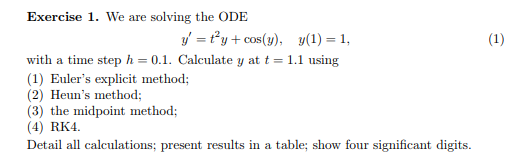Exercise 1. We are solving the ODE yycos)1) 1, with a time step h 0.1. Calculate y at t-1.1 using (1) Euler's explicit method; Helu (3) the midpoint method; (A) RKA Detail all calculations; present results in a table; show four significant digits eun's metho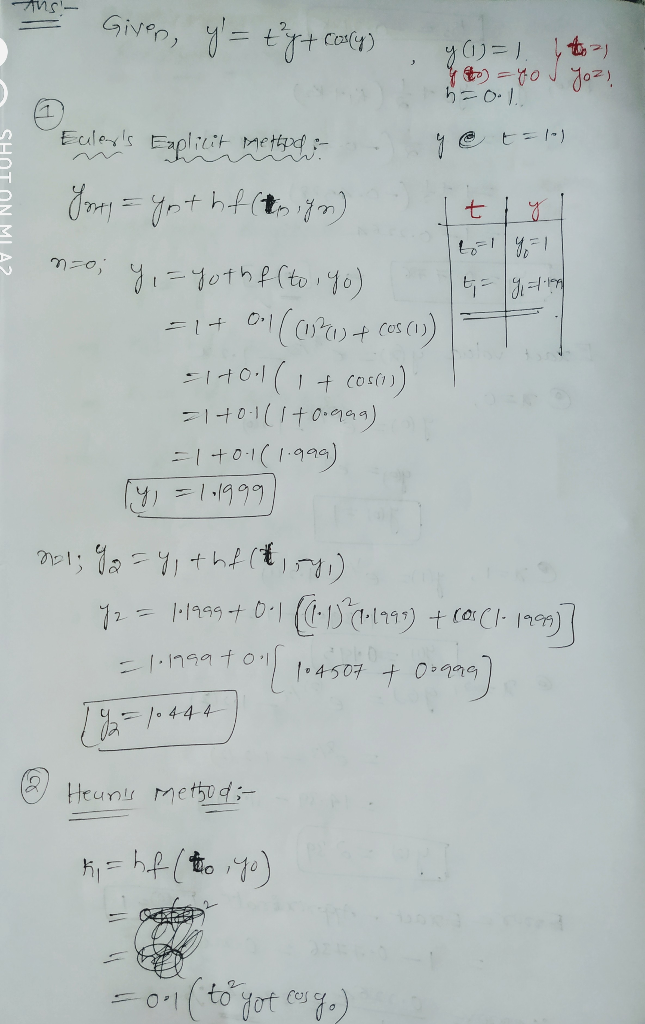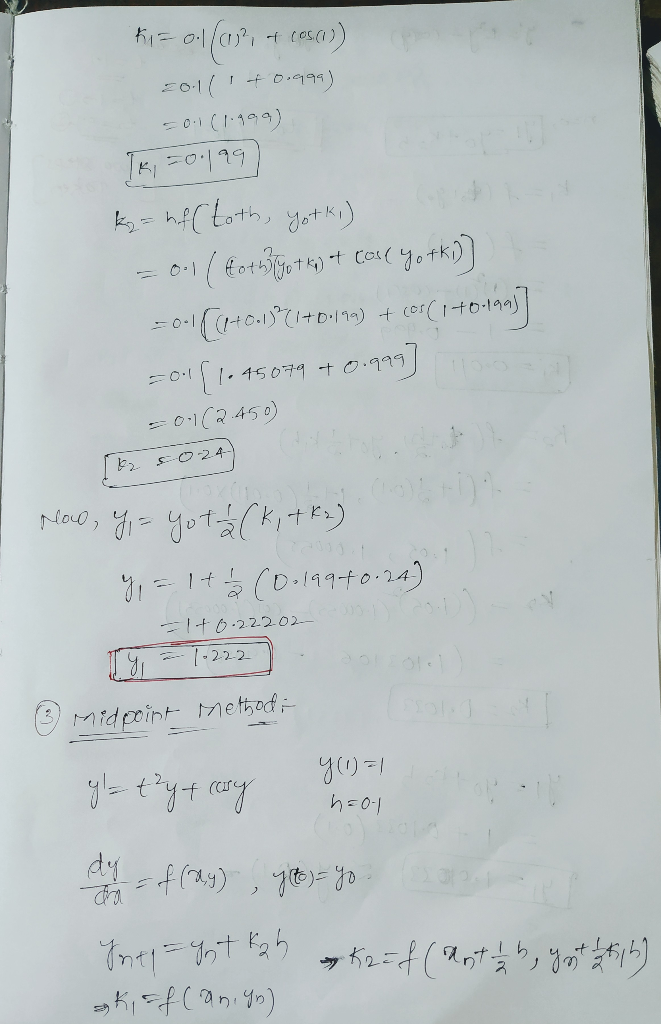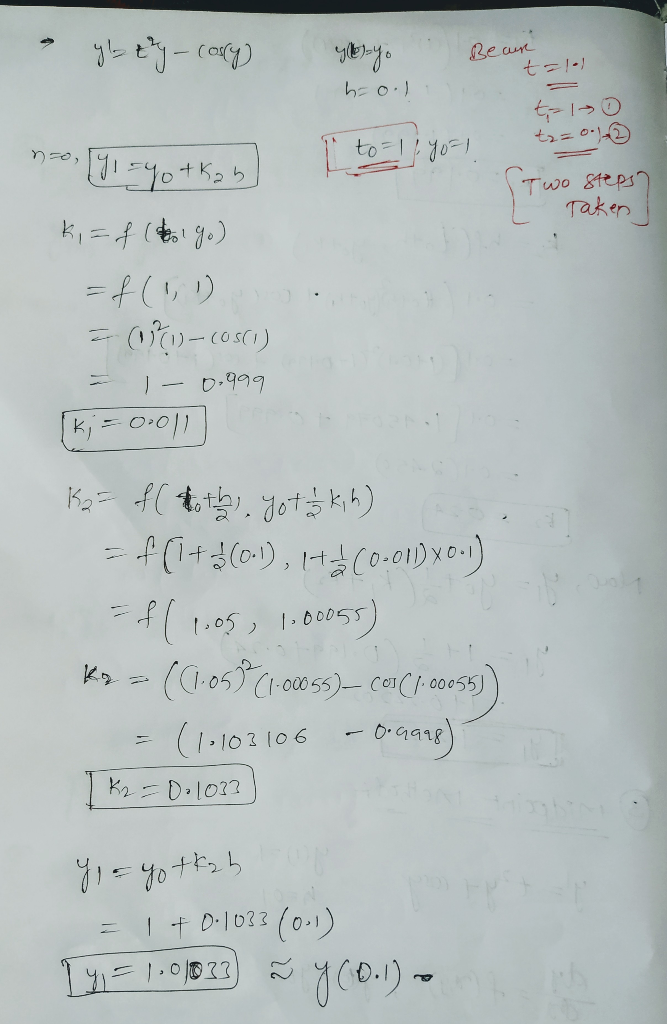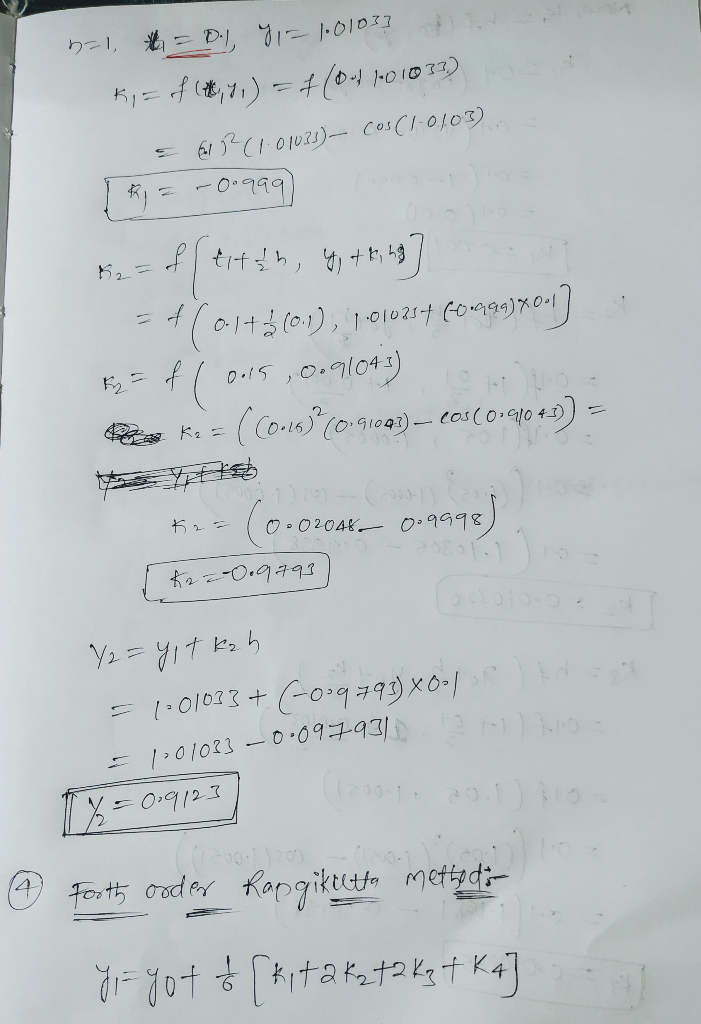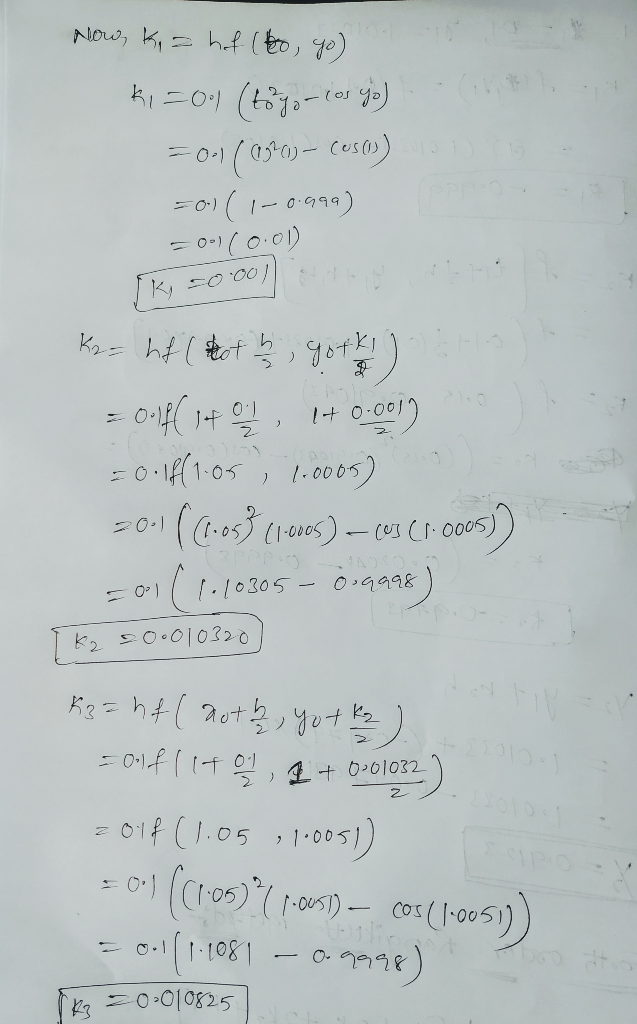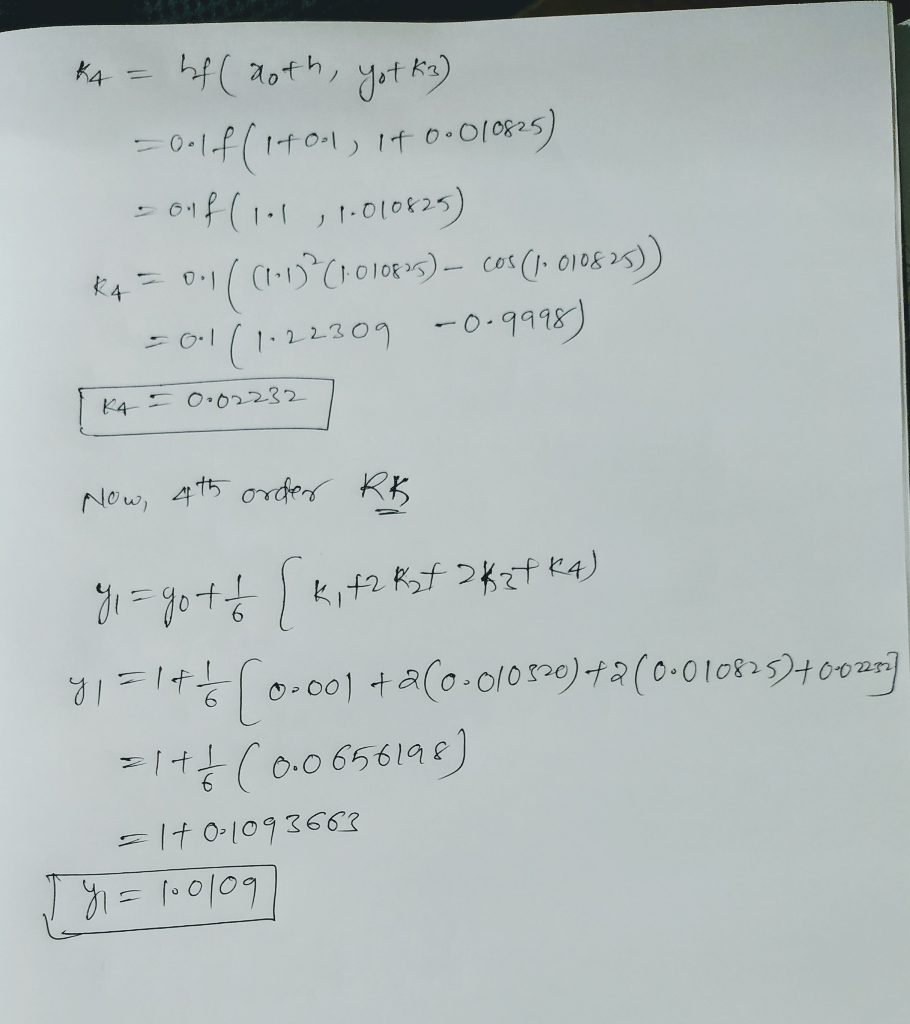If any doubt just comment . Thanks

Add Answer of: Exercise 1. We are solving the ODE yycos)1) 1, with a time step h 0.1. Calculate y at t-1.1 using...
More Homework Help Questions Additional questions in this topic.

• Second order systems of ordinary differential equations (ODE) often describe motional systems involving multiple masses. Solve the following second order system of ODE using Laplace transform meth...

Need Online Homework Help?

Get FREE EXPERT Answers
WITHIN MINUTES
Related Questions